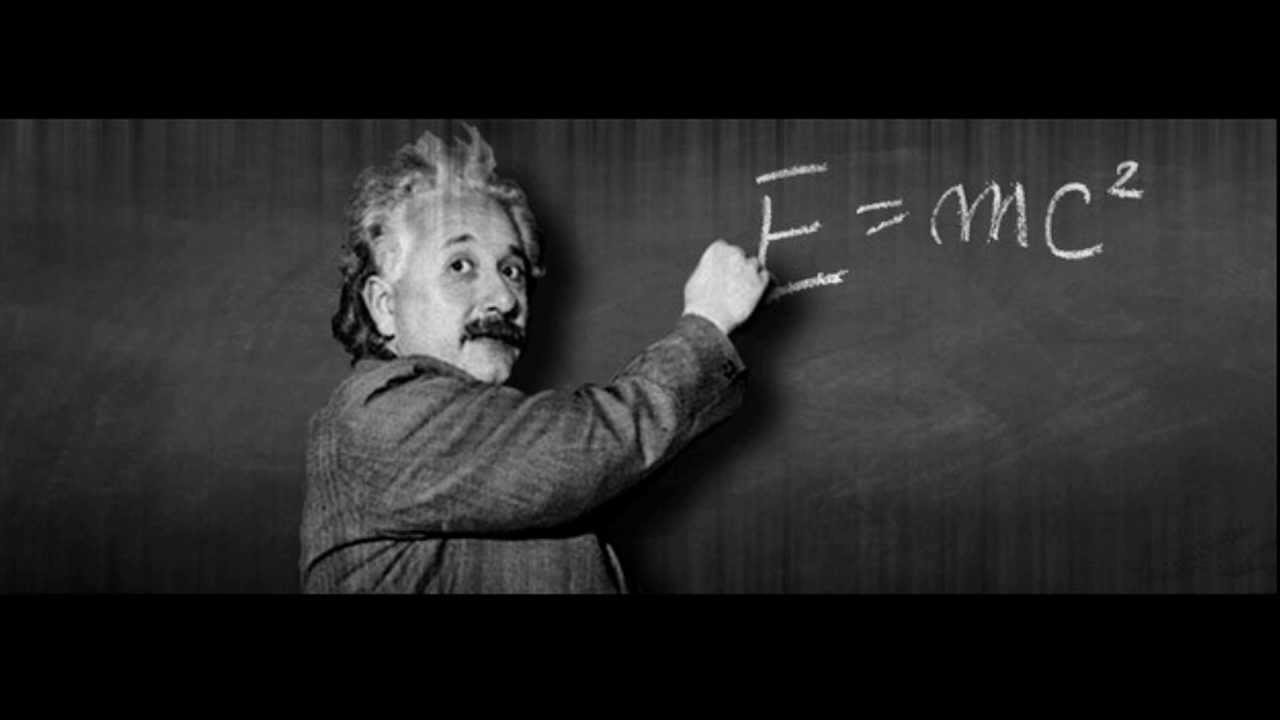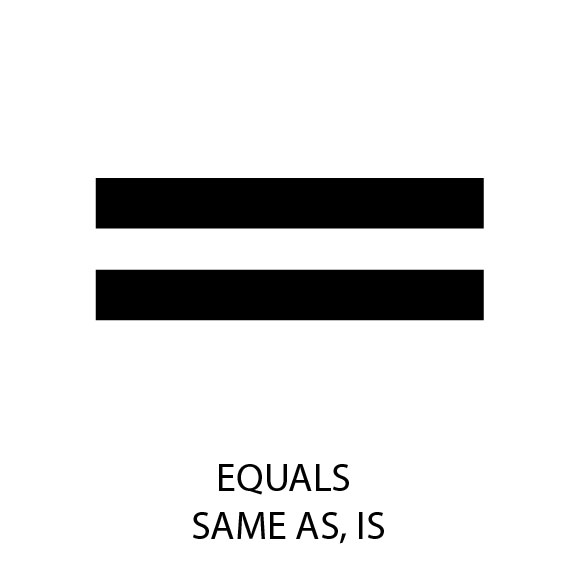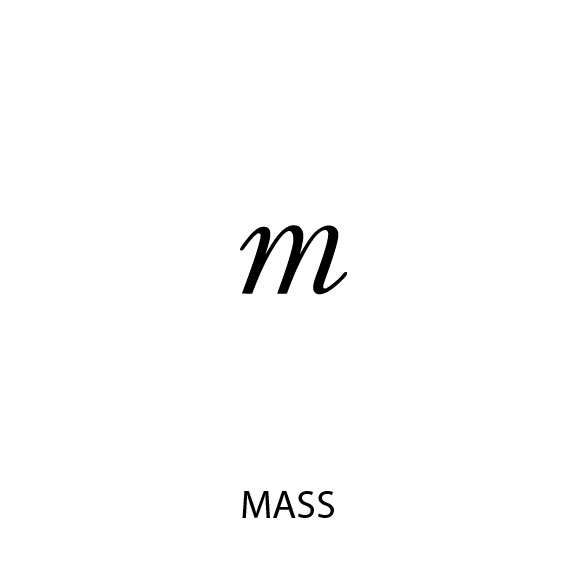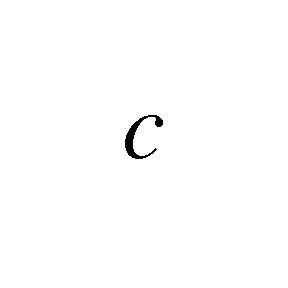# E equals mc2Conventional

E=mc2

Einstein's famous equation of 1905 describes expansive entropic Energy derived from mass accelerated. It only describes this dispersive energy and mentions nothing about equated syntropic Force. Hence the equation reflects mass accelerated and not the liberation of energy from this balanced condition of a mass.

Lack of Definitions The speed of light is held (in this equation) to be a constant whereas it is well known the speed of light is dependent upon the media through which it passes. As there is no absolutely empty vacuum all available vacuous media have some undetermined density. Until and unless this density is stipulated the speed of light is in question. Similarly, neither Energy nor mass are defined.

"There is no dividing of matter and force into two distinct terms, as they both are ONE. FORCE is liberated matter. MATTER is force in bondage." [Keely, 1893] "Matter is bound up energy and energy is liberated matter." [Keely, 1893]

Russell "This eternal fact of Nature has always stared man in the face very conspicuously, without having been seen. Even so great a thinker and observer as Albert Einstein passed it by when he wrote his equation of 1905, which states that fast motion multiplies electric potential. That fact of Nature is true, but as it is equally true that fast motion divides potential his whole equation is invalid as a law, for a half truth which voids itself cannot become an eternal law." [Atomic Suicide, page 21]

"One half of His Law of Love has been fulfilled. Up to that point The Einstein Equation of 1905 fits perfectly. It fully accounts for the mathematics of life, but not for death. The other half of the Law of Love must now be fulfilled. That which has been given must be equally regiven." [Atomic Suicide, page 36]

"CONSTRUCTION OF ONE CYCLE OF AN ELECTRIC CURRENT Electric Potential Multiplies in accord with Einstein Equation

Electric Potential divides Einstein Equation now reverses" [Atomic Suicide, page 163]

"The Einstein Equation properly defined this principle by the words which say that fast motion multiplies electric potential, but that principle has not been applied to the mechanics of coil making. For that reason the loops of force which cylindrical coils create, are not focused as they would be if they followed the crystalline shape of the balanced electric current as shown in Fig. 70." [Atomic Suicide, page 273]In SVP mass is considered to be Force and Energy balanced in a Latent state or simply Latent Force. All mass contains Latent Force that can be liberated or released. This balanced state or condition can be destabilized or unbalanced thus releasing or liberating its bound up forces manifesting as radiant or dispersive energies. [See Disturbance of Equilibrium]